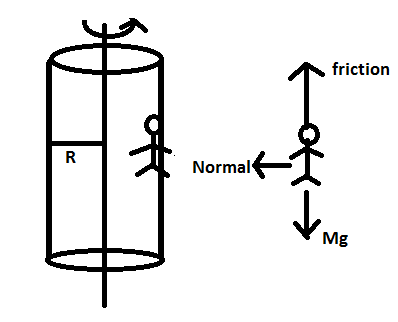# a 70 kg man leans against a hollow cylindrical wall with radius 3m.coefficient of friction between clothing and wall is 0.15.find minimum speed of rotation of cylinder so that the man doesnt fall when the floor is removed?

9 years agoTaking the man to be the system,

Normal force supplies the necessary centripetal force.

therefore, N=mv2/R

Also, to ensure that the person does not fall, frictional force should be equal to the person''s weight.

therefore, F= mg

=> µs.N=mg

from (1)

µs.mv2/R=mg

µs(Rω)2/R=g                 {v=Rω}

(0.15)(3)(ω)2 =10

ω2= 10/0.45= 22.22

ω = 4.71 rad/sec2

Hope it helps. Do approve if satisfied by the answer!

All the best!